Successfully reported this slideshow.
We use your LinkedIn profile and activity data to personalize ads and to show you more relevant ads. You can change your ad preferences anytime.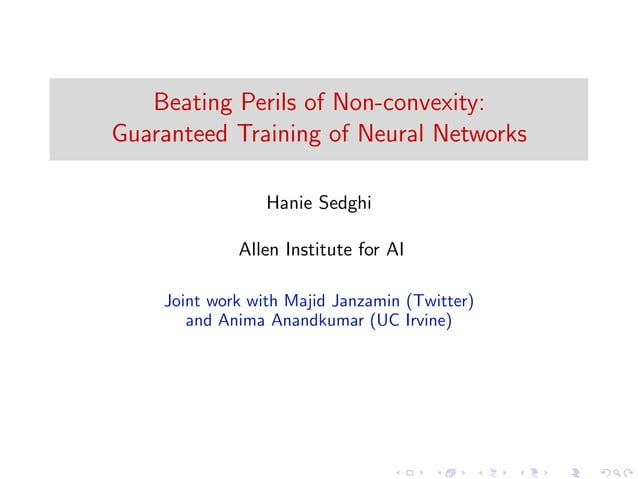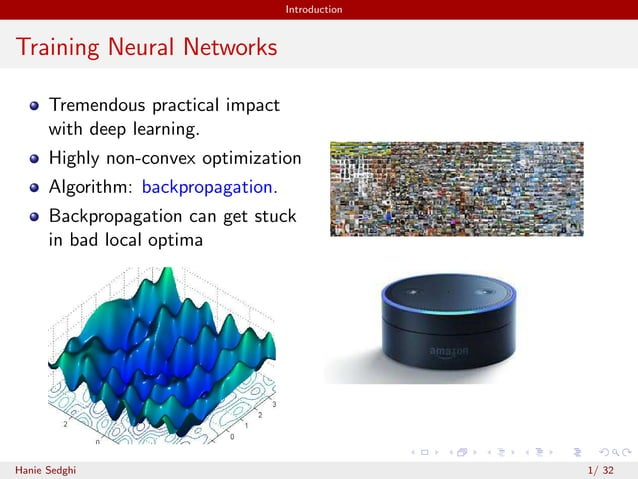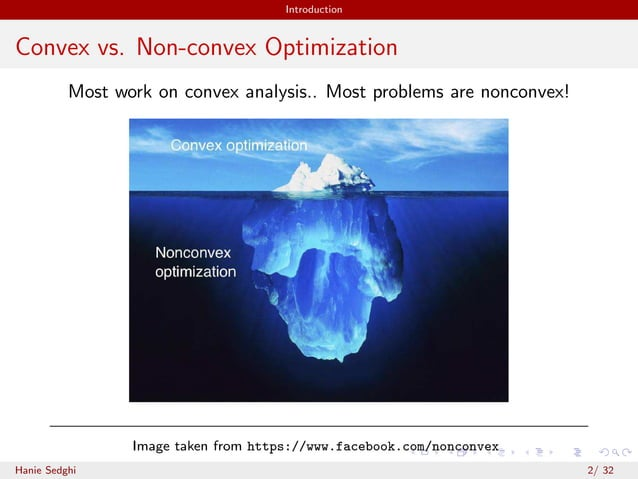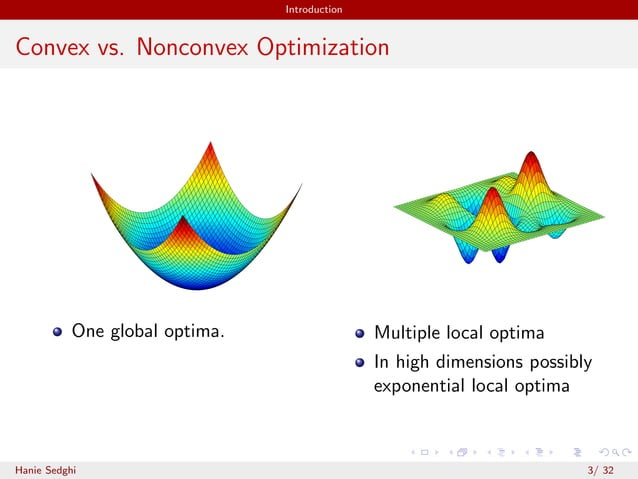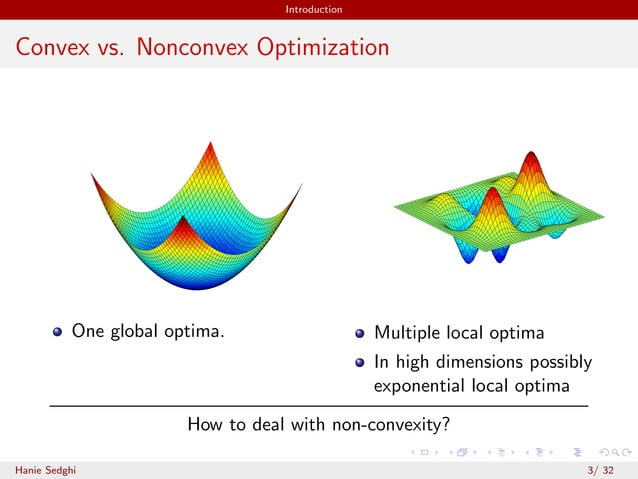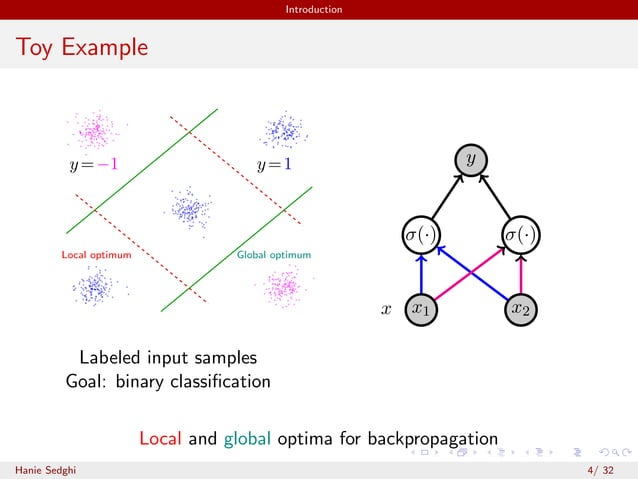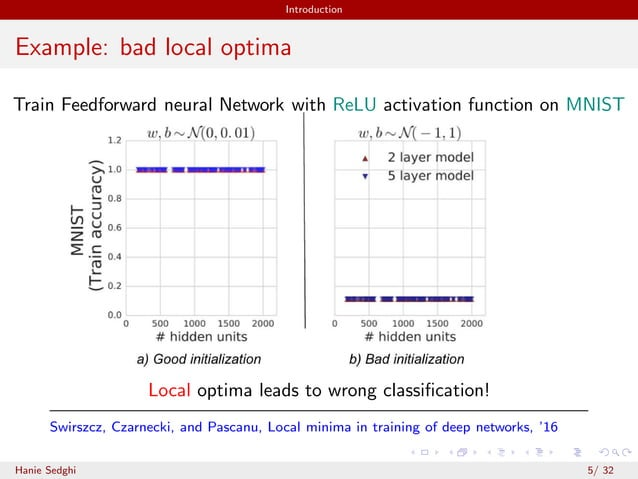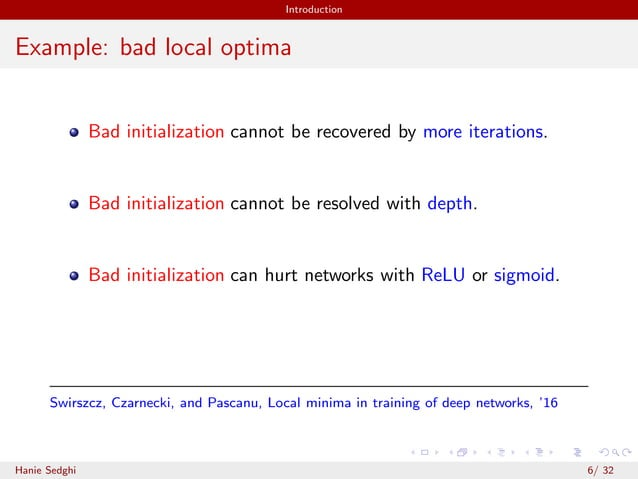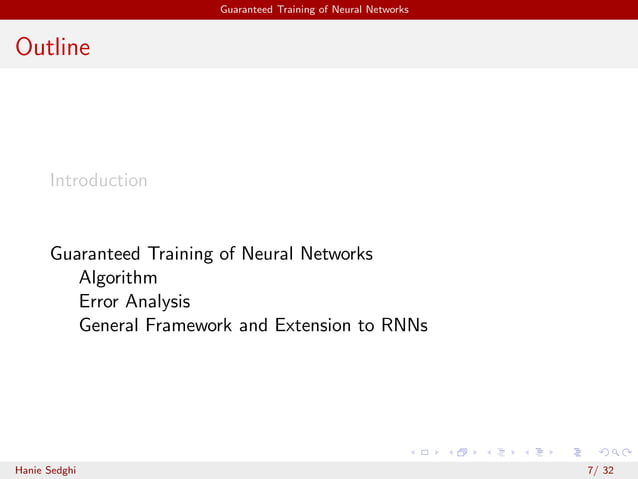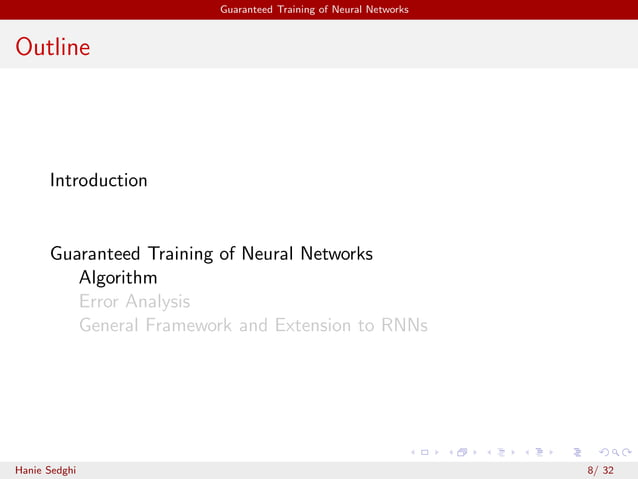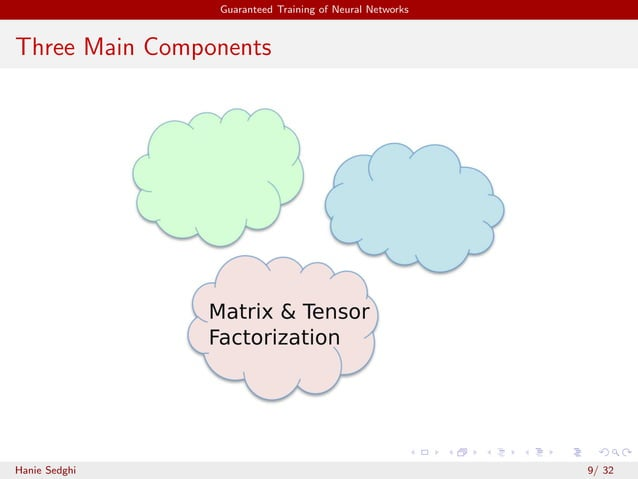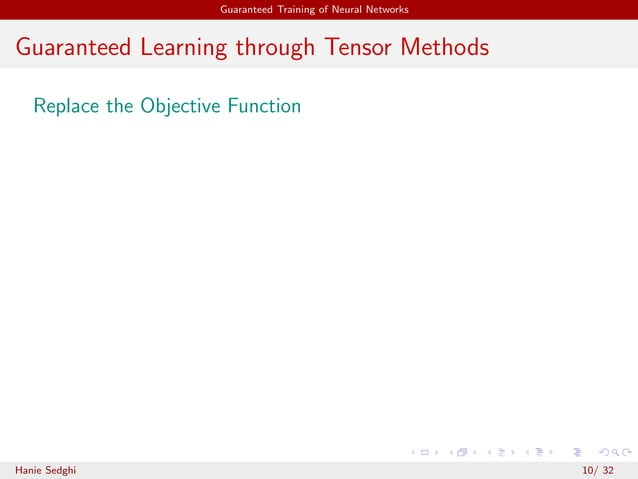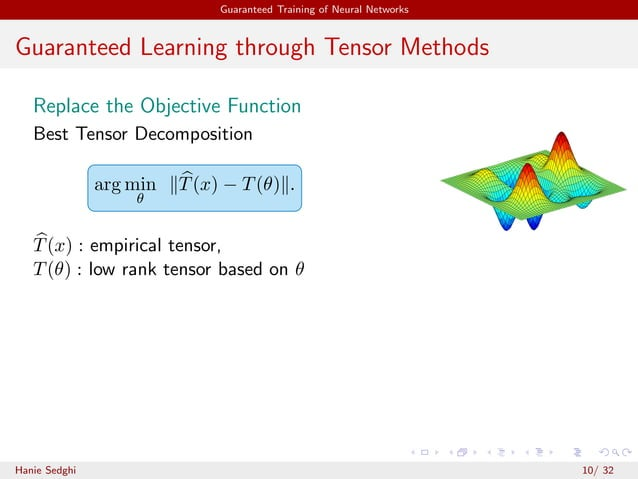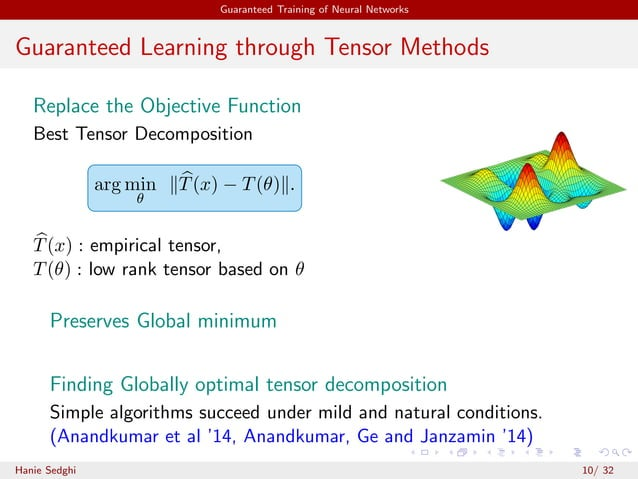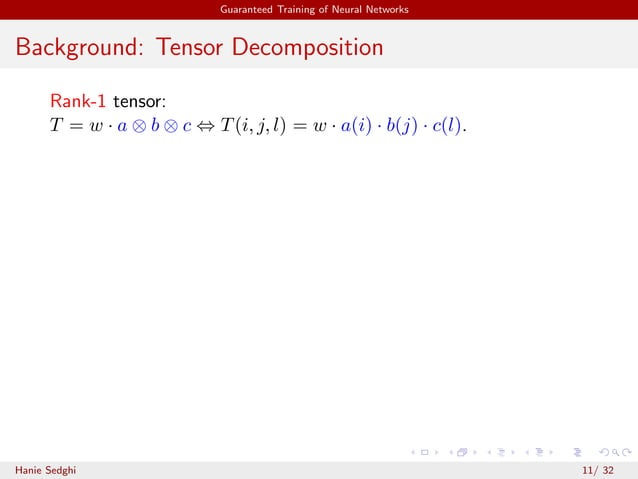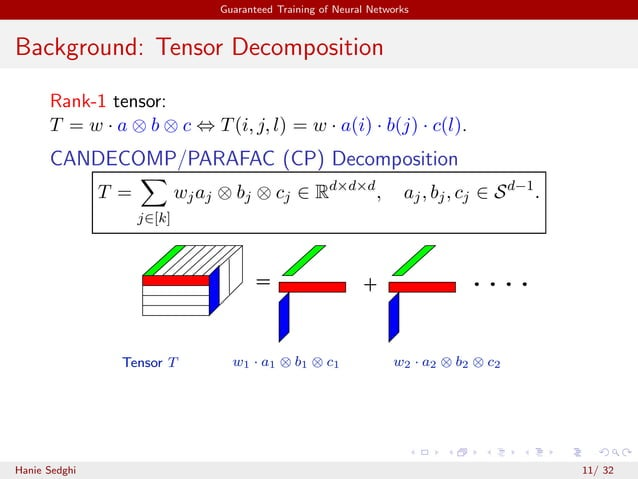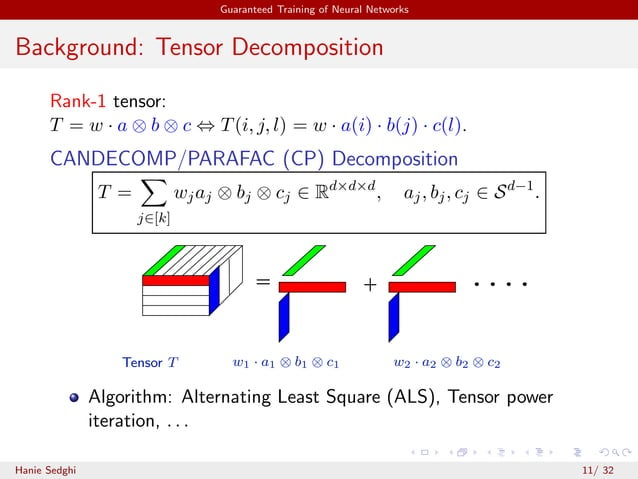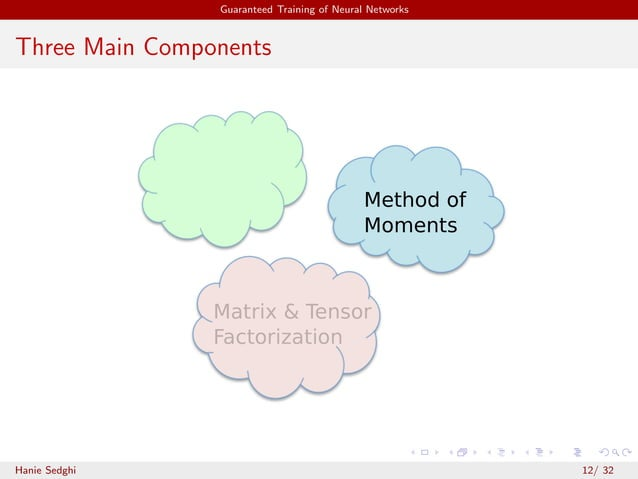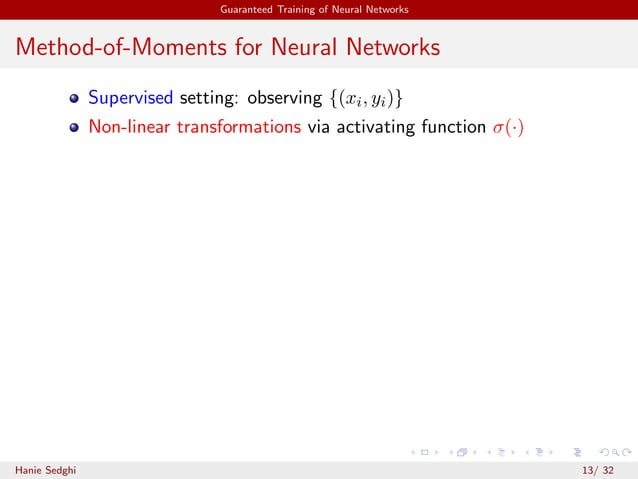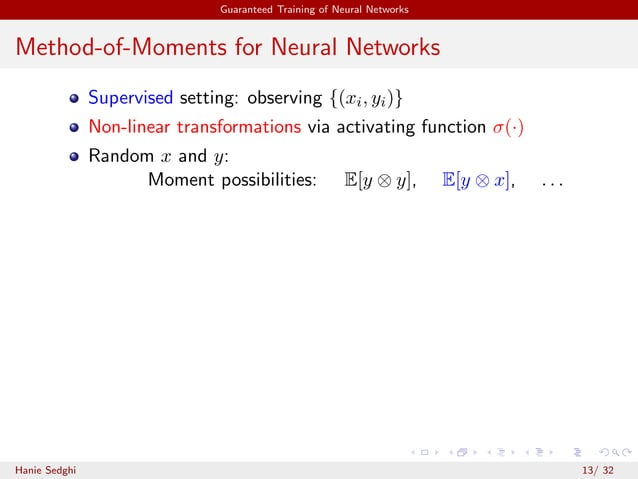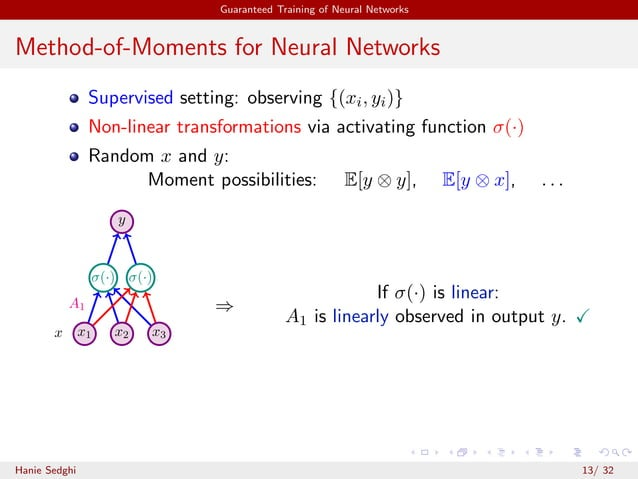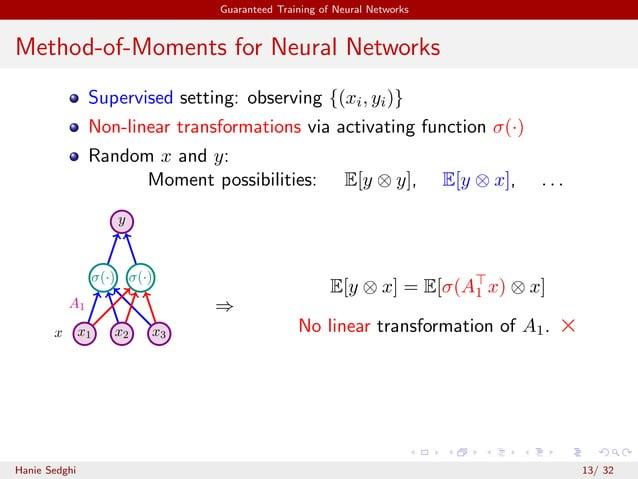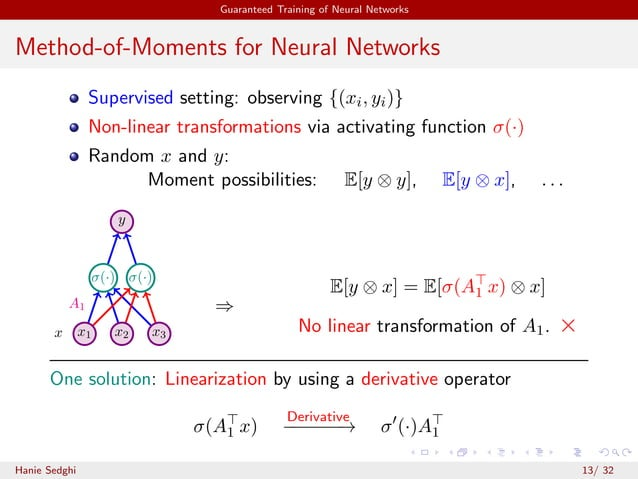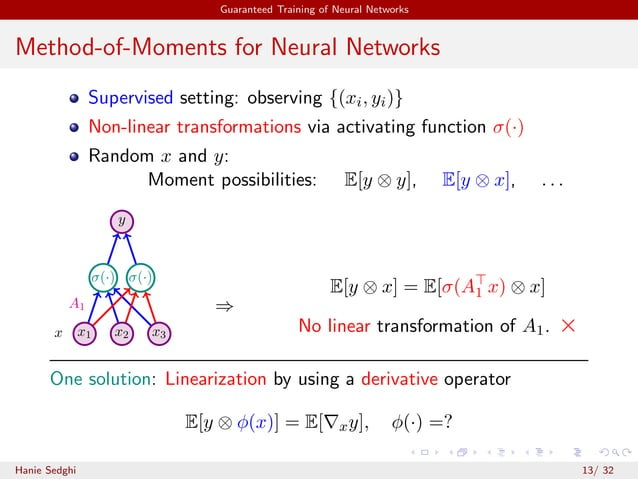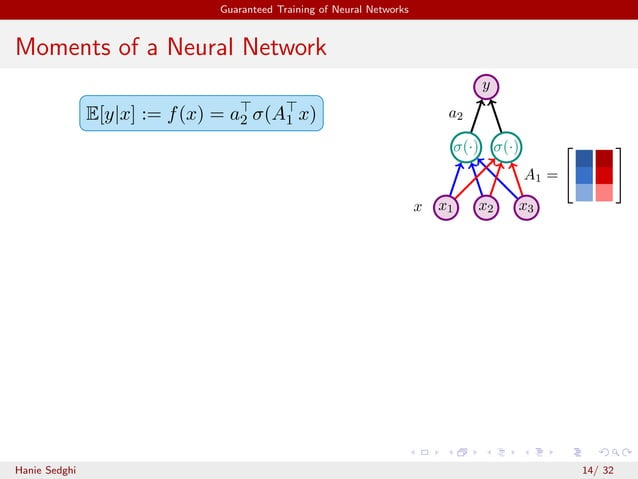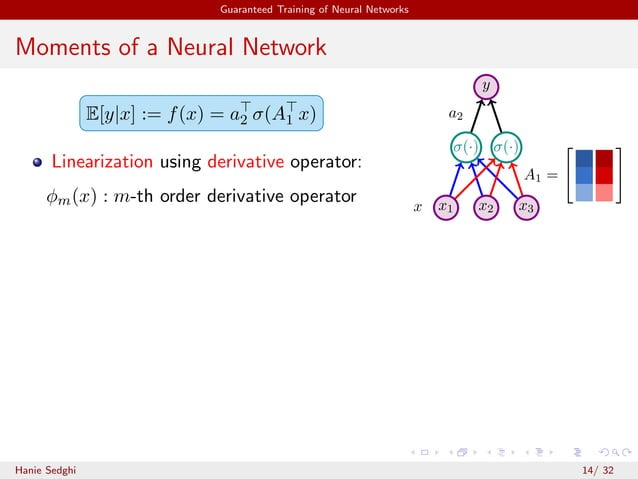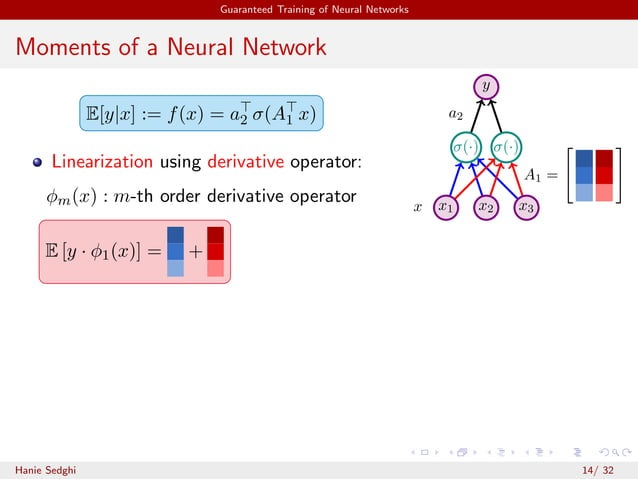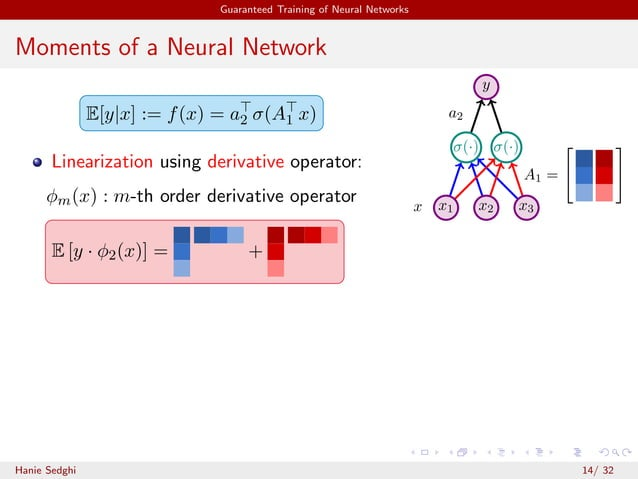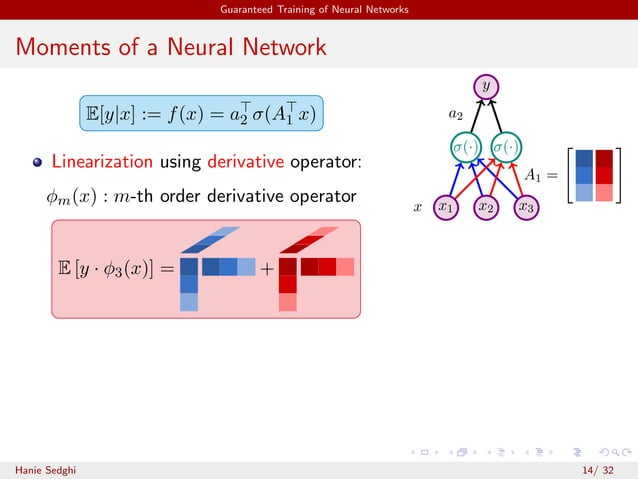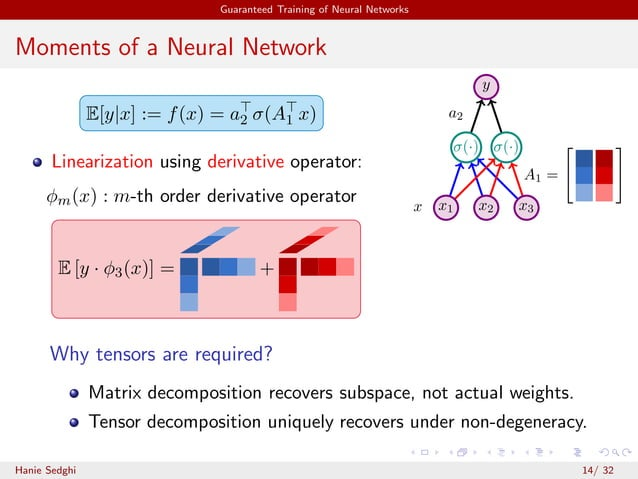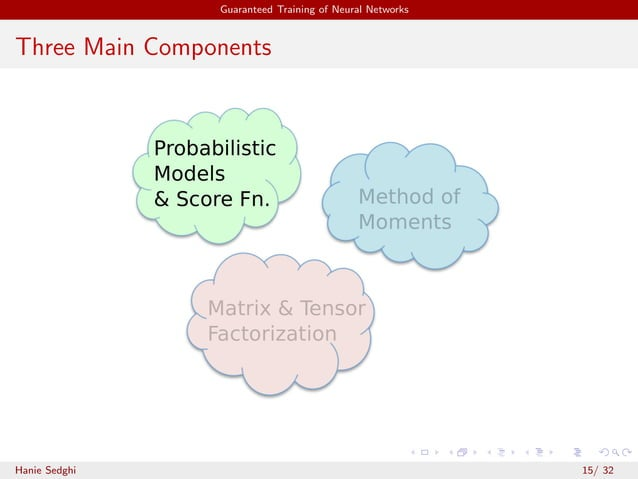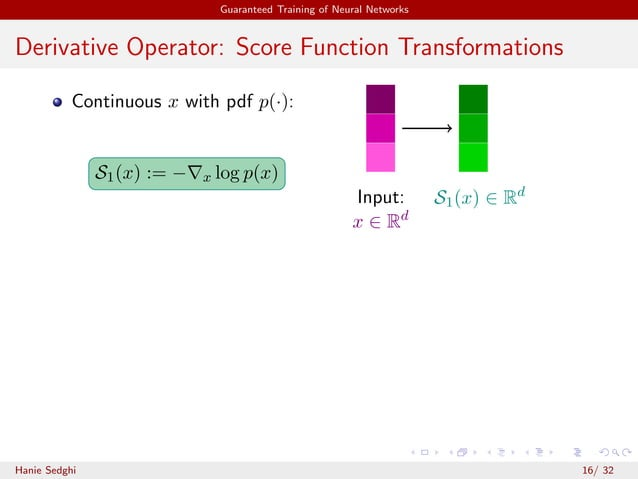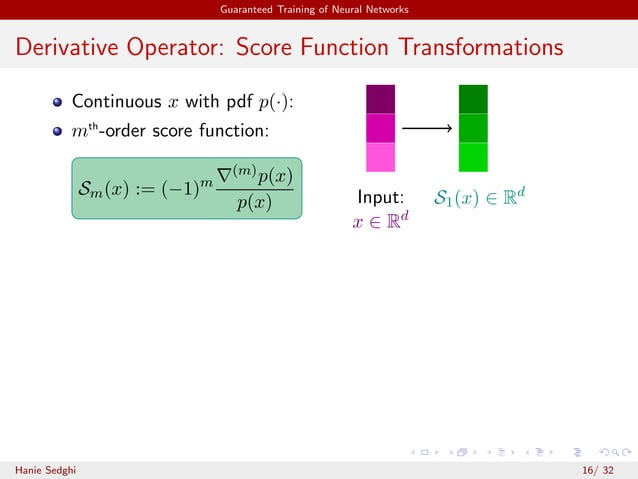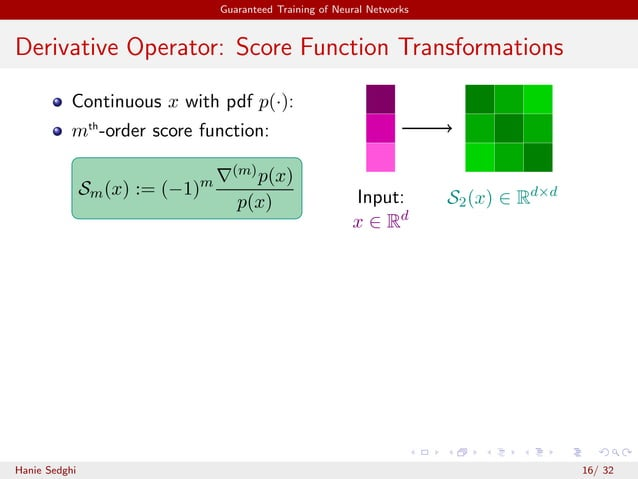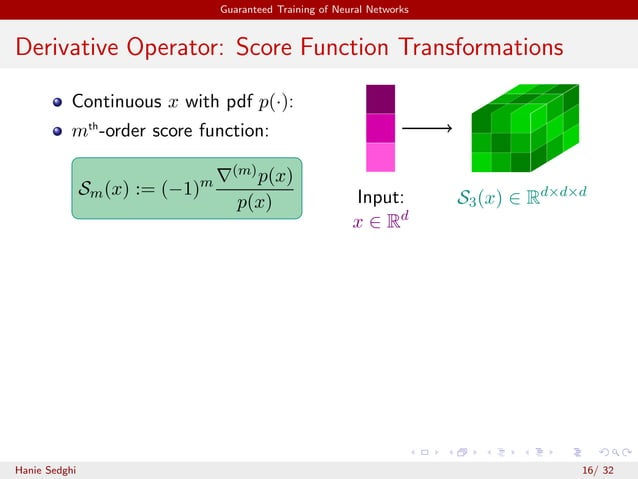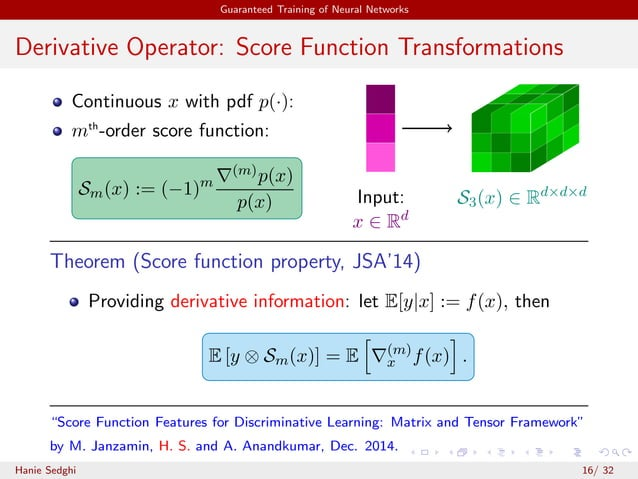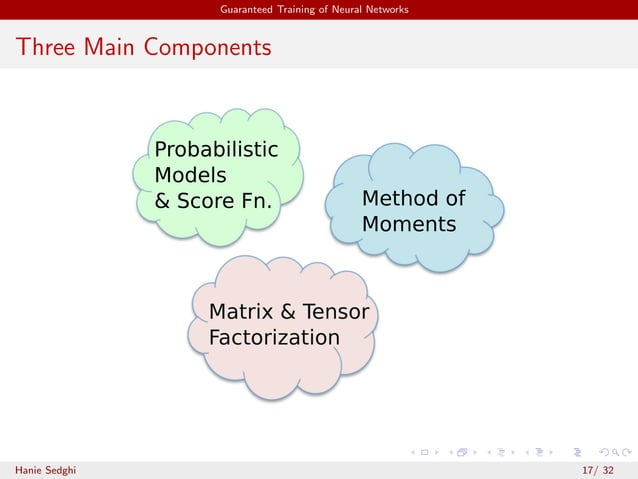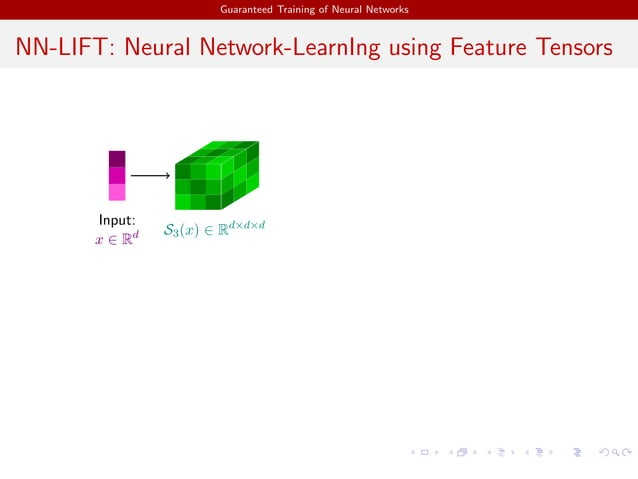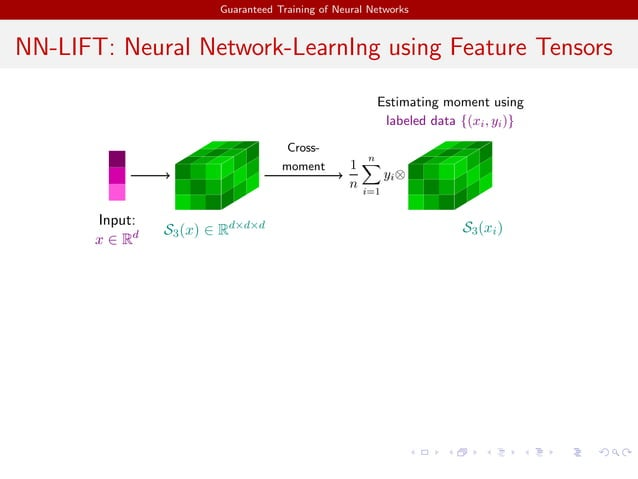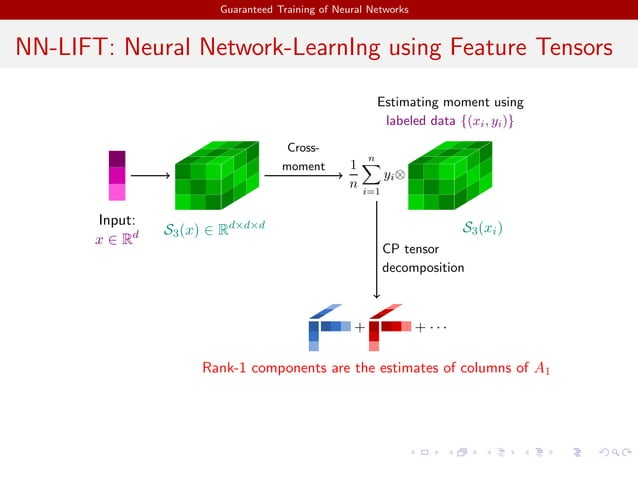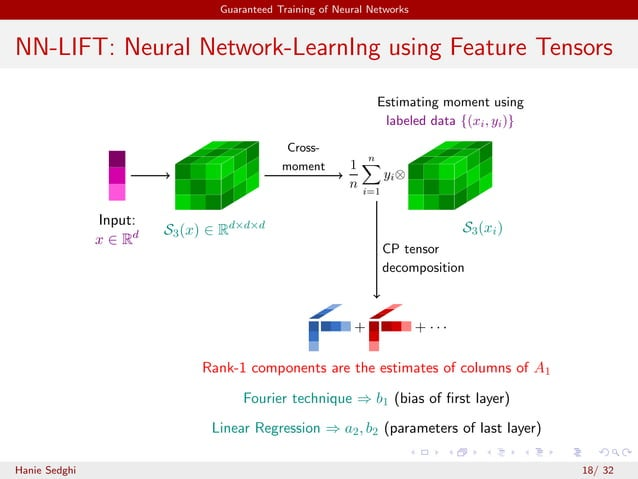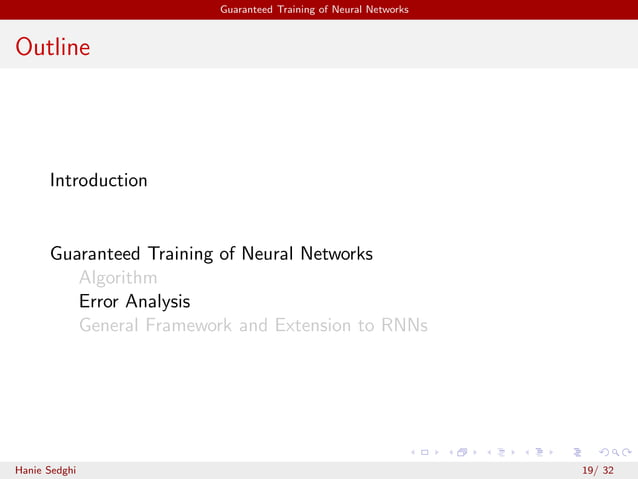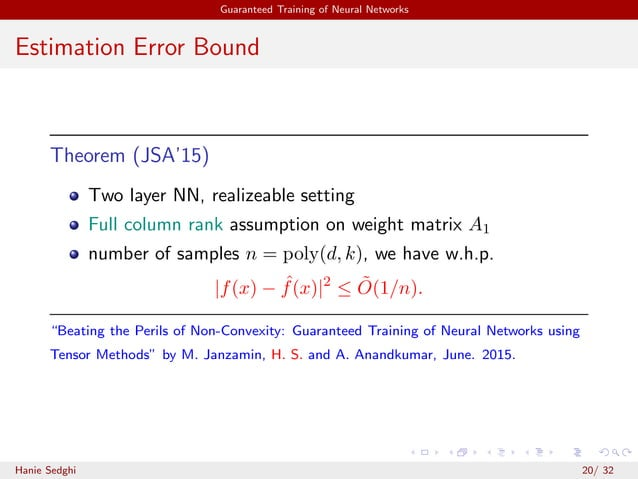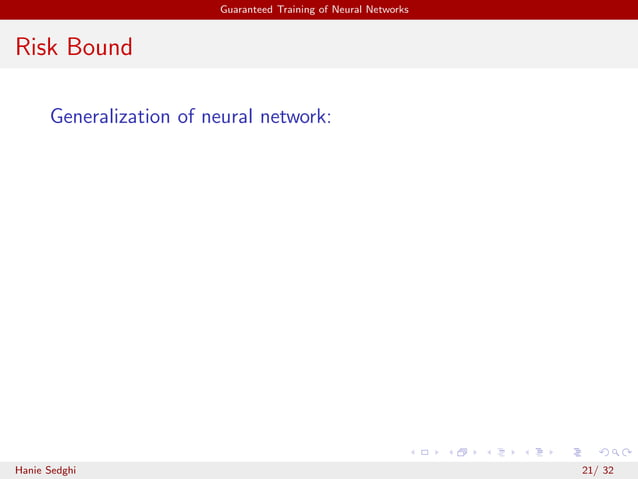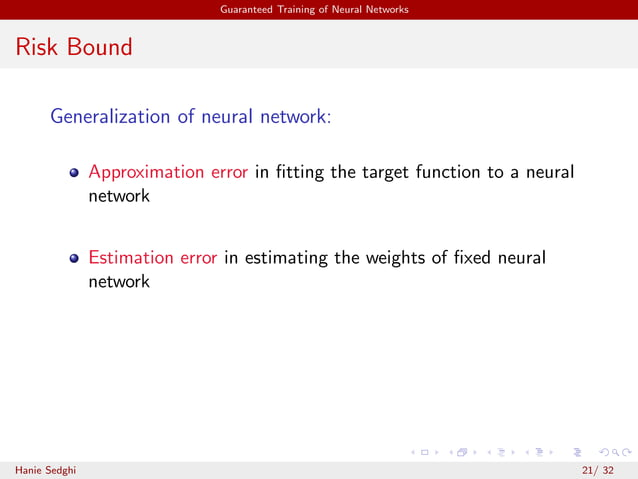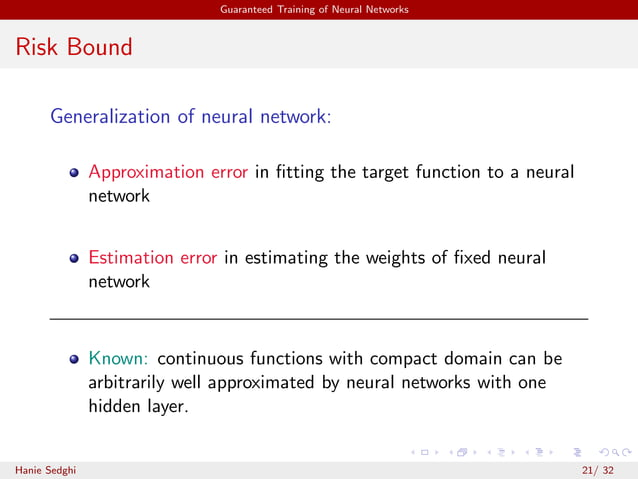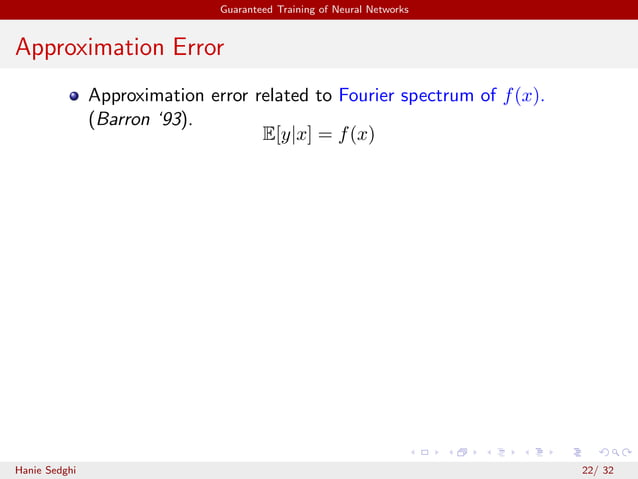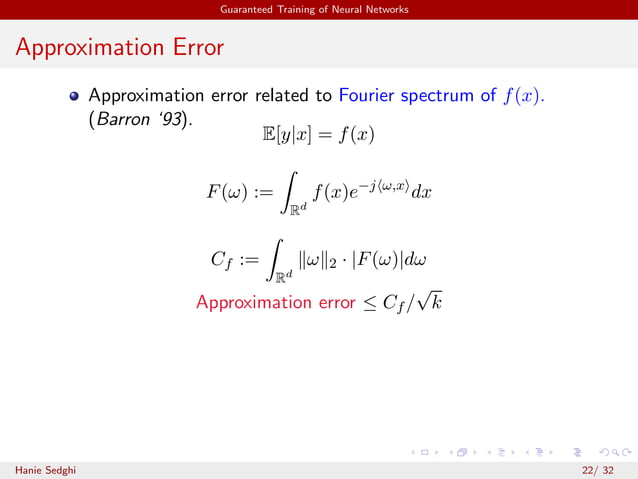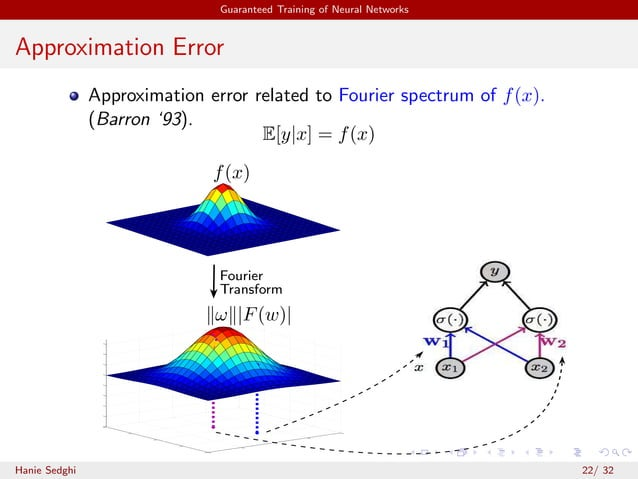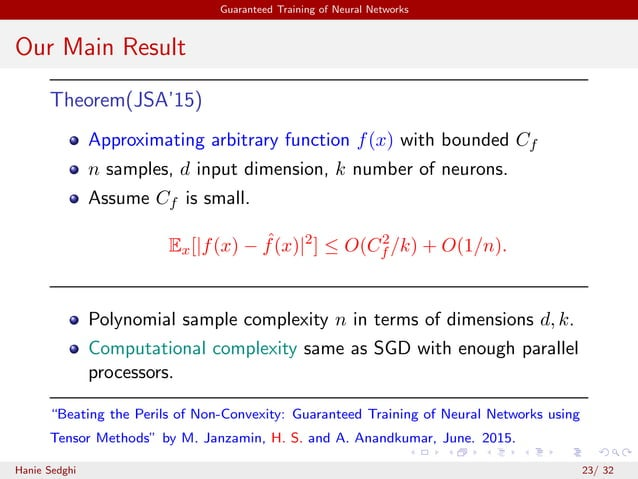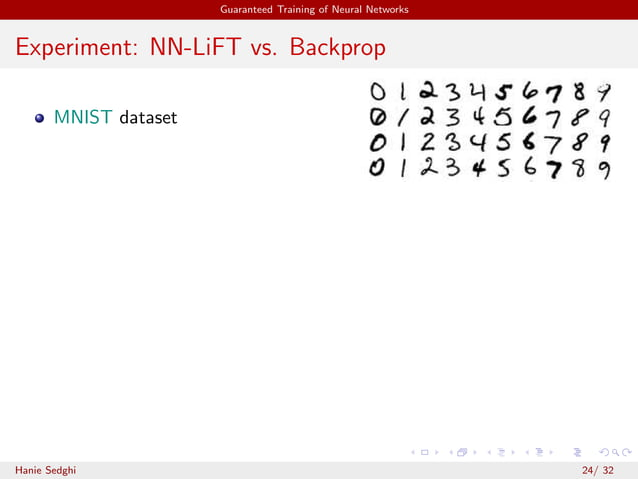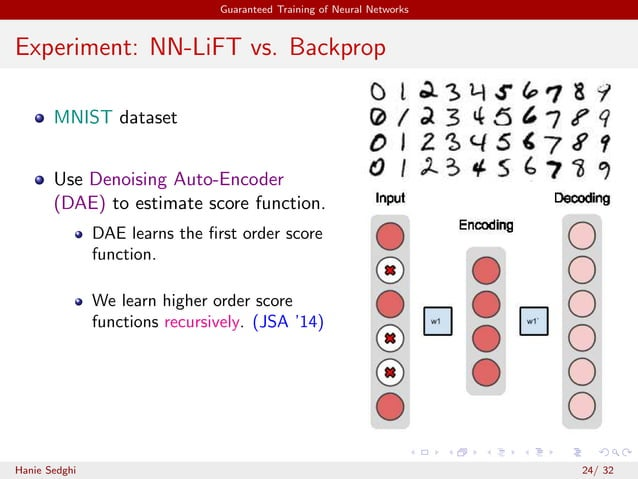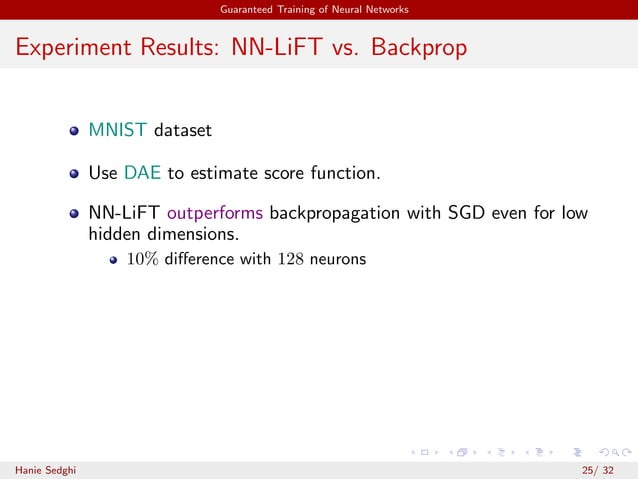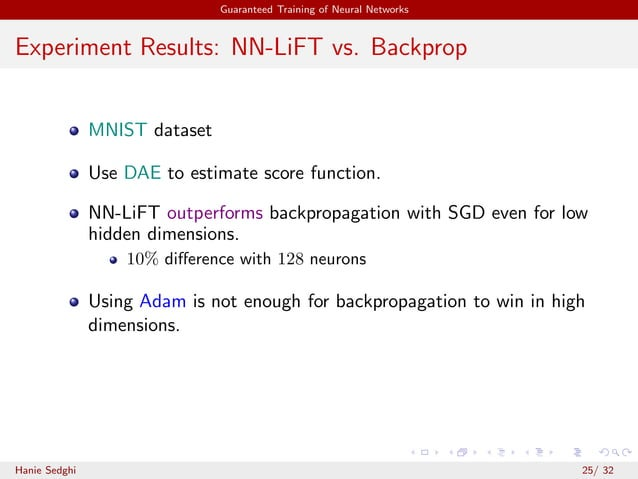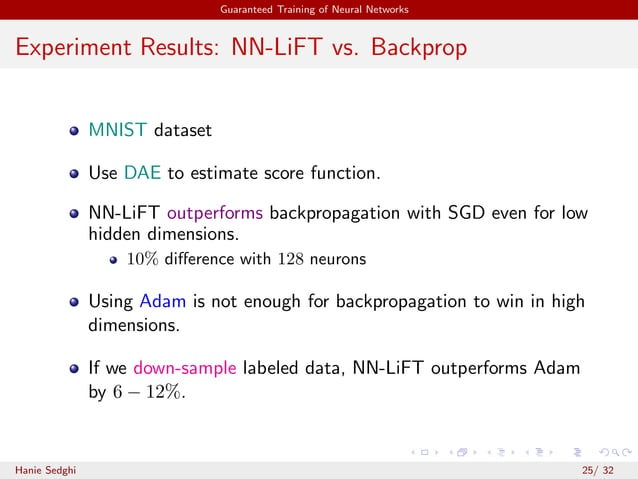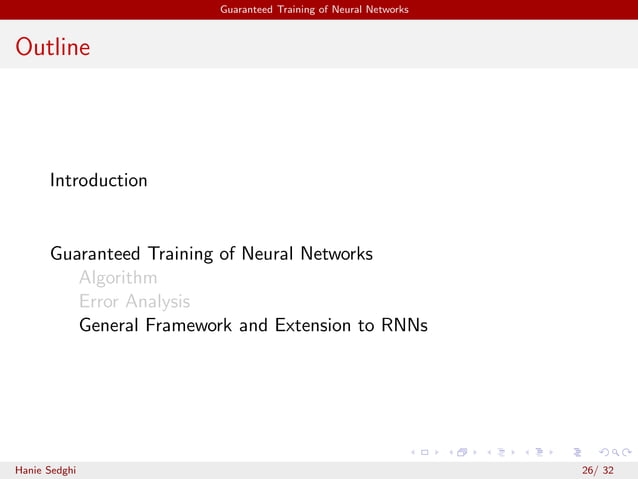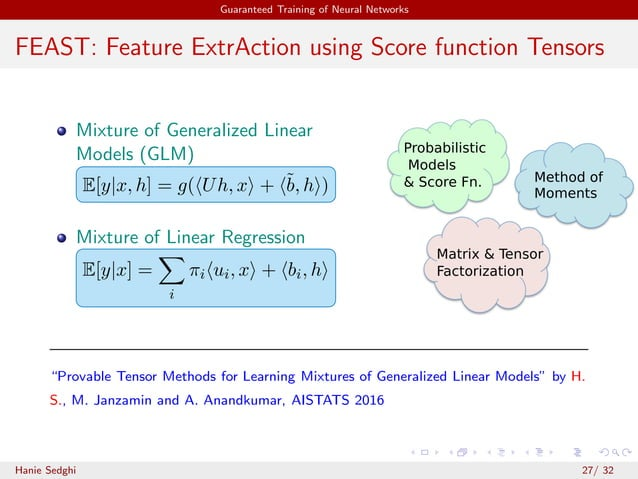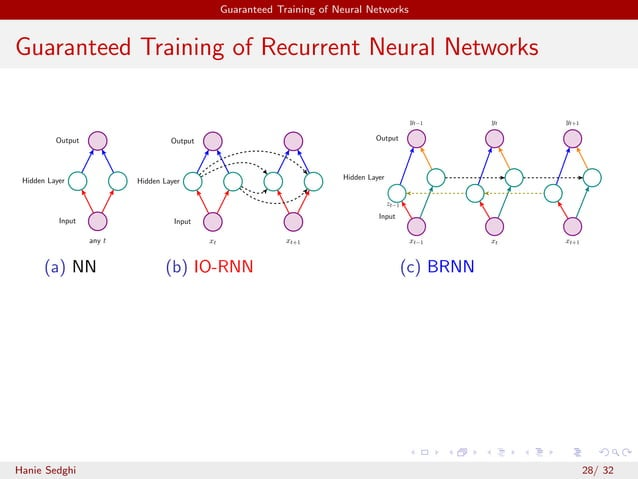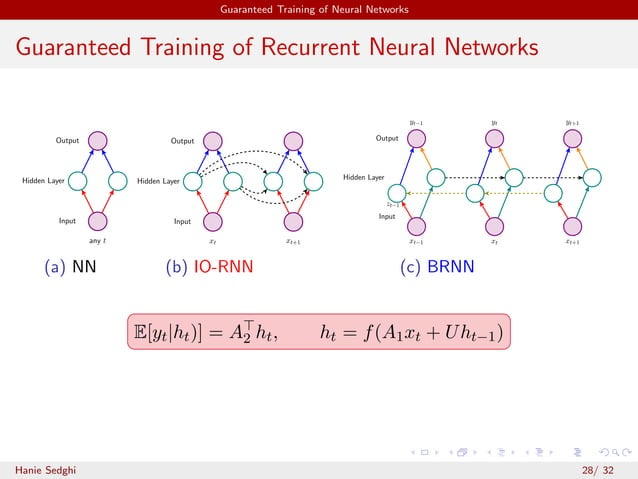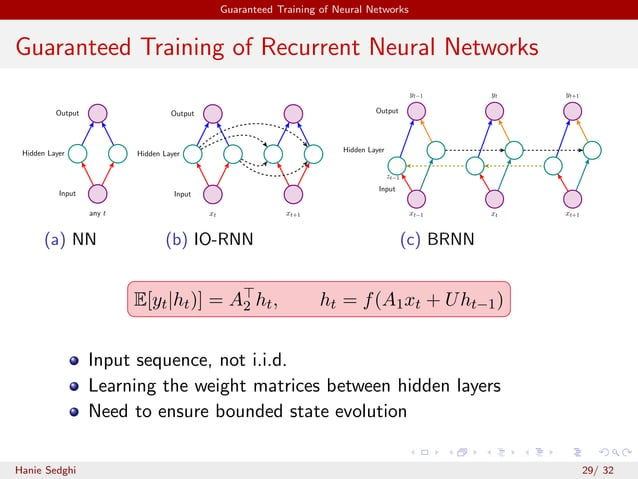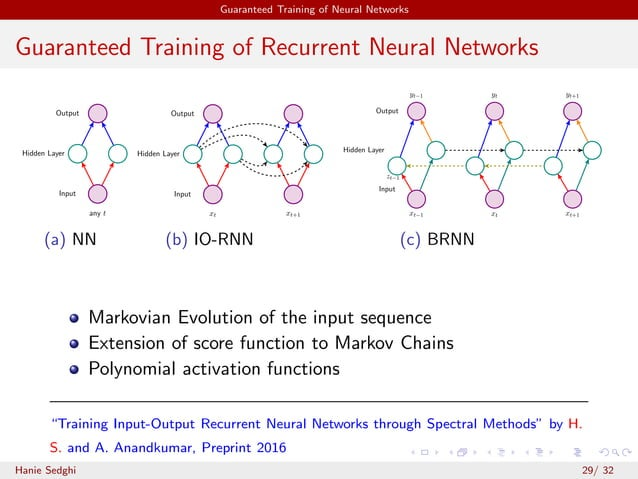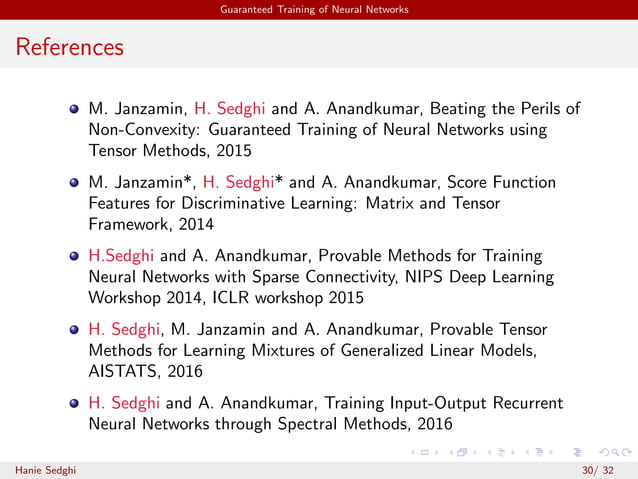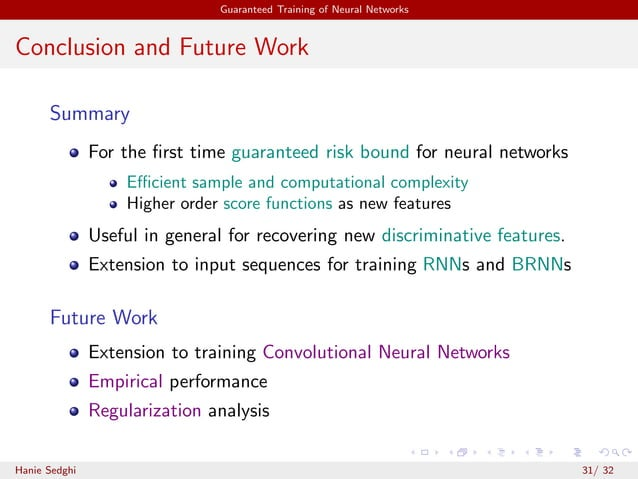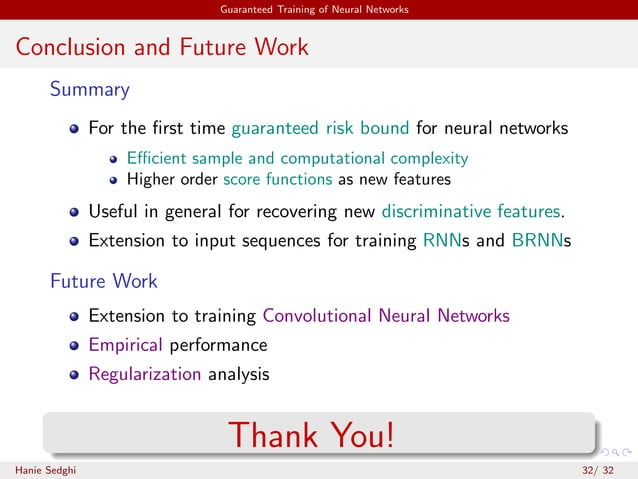Upcoming SlideShare
Loading in …5
×

# Hanie Sedghi, Research Scientist at Allen Institute for Artificial Intelligence, at MLconf Seattle 2017

Hanie Sedghi is a Research Scientist at Allen Institute for Artificial Intelligence (AI2). Her research interests include large-scale machine learning, high-dimensional statistics and probabilistic models. More recently, she has been working on inference and learning in latent variable models. She has received her Ph.D. from University of Southern California with a minor in Mathematics in 2015. She was also a visiting researcher at University of California, Irvine working with professor Anandkumar during her Ph.D. She received her B.Sc. and M.Sc. degree from Sharif University of Technology, Tehran, Iran.

Abstract summary

Beating Perils of Non-convexity:Guaranteed Training of Neural Networks using Tensor Methods:
Neural networks have revolutionized performance across multiple domains such as computer vision and speech recognition. However, training a neural network is a highly non-convex problem and the conventional stochastic gradient descent can get stuck in spurious local optima. We propose a computationally efficient method for training neural networks that also has guaranteed risk bounds. It is based on tensor decomposition which is guaranteed to converge to the globally optimal solution under mild conditions. We explain how this framework can be leveraged to train feedforward and recurrent neural networks.

• Full Name
Comment goes here.

Are you sure you want to Yes No
Your message goes here• Be the first to comment

• Be the first to like this

### Hanie Sedghi, Research Scientist at Allen Institute for Artificial Intelligence, at MLconf Seattle 2017

1. 1. Beating Perils of Non-convexity: Guaranteed Training of Neural Networks Hanie Sedghi Allen Institute for AI Joint work with Majid Janzamin (Twitter) and Anima Anandkumar (UC Irvine)
2. 2. Introduction Training Neural Networks Tremendous practical impact with deep learning. Highly non-convex optimization Algorithm: backpropagation. Backpropagation can get stuck in bad local optima Hanie Sedghi 1/ 32
3. 3. Introduction Convex vs. Non-convex Optimization Most work on convex analysis.. Most problems are nonconvex! Image taken from https://www.facebook.com/nonconvex Hanie Sedghi 2/ 32
4. 4. Introduction Convex vs. Nonconvex Optimization One global optima. Multiple local optima In high dimensions possibly exponential local optima Hanie Sedghi 3/ 32
5. 5. Introduction Convex vs. Nonconvex Optimization One global optima. Multiple local optima In high dimensions possibly exponential local optima How to deal with non-convexity? Hanie Sedghi 3/ 32
6. 6. Introduction Toy Example y=1y=−1 Local optimum Global optimum Labeled input samples Goal: binary classiﬁcation σ(·) σ(·) y x1 x2x Local and global optima for backpropagation Hanie Sedghi 4/ 32
7. 7. Introduction Example: bad local optima Train Feedforward neural Network with ReLU activation function on MNIST Local optima leads to wrong classiﬁcation! Swirszcz, Czarnecki, and Pascanu, Local minima in training of deep networks, ’16 Hanie Sedghi 5/ 32
8. 8. Introduction Example: bad local optima Bad initialization cannot be recovered by more iterations. Bad initialization cannot be resolved with depth. Bad initialization can hurt networks with ReLU or sigmoid. Swirszcz, Czarnecki, and Pascanu, Local minima in training of deep networks, ’16 Hanie Sedghi 6/ 32
9. 9. Guaranteed Training of Neural Networks Outline Introduction Guaranteed Training of Neural Networks Algorithm Error Analysis General Framework and Extension to RNNs Hanie Sedghi 7/ 32
10. 10. Guaranteed Training of Neural Networks Outline Introduction Guaranteed Training of Neural Networks Algorithm Error Analysis General Framework and Extension to RNNs Hanie Sedghi 8/ 32
11. 11. Guaranteed Training of Neural Networks Three Main Components Hanie Sedghi 9/ 32
12. 12. Guaranteed Training of Neural Networks Guaranteed Learning through Tensor Methods Replace the Objective Function Hanie Sedghi 10/ 32
13. 13. Guaranteed Training of Neural Networks Guaranteed Learning through Tensor Methods Replace the Objective Function Best Tensor Decomposition arg min θ T(x) − T(θ) . T(x) : empirical tensor, T(θ) : low rank tensor based on θ Hanie Sedghi 10/ 32
14. 14. Guaranteed Training of Neural Networks Guaranteed Learning through Tensor Methods Replace the Objective Function Best Tensor Decomposition arg min θ T(x) − T(θ) . T(x) : empirical tensor, T(θ) : low rank tensor based on θ Preserves Global minimum Finding Globally optimal tensor decomposition Simple algorithms succeed under mild and natural conditions. (Anandkumar et al ’14, Anandkumar, Ge and Janzamin ’14) Hanie Sedghi 10/ 32
15. 15. Guaranteed Training of Neural Networks Background: Tensor Decomposition Rank-1 tensor: T = w · a ⊗ b ⊗ c ⇔ T(i, j, l) = w · a(i) · b(j) · c(l). Hanie Sedghi 11/ 32
16. 16. Guaranteed Training of Neural Networks Background: Tensor Decomposition Rank-1 tensor: T = w · a ⊗ b ⊗ c ⇔ T(i, j, l) = w · a(i) · b(j) · c(l). CANDECOMP/PARAFAC (CP) Decomposition T = j∈[k] wjaj ⊗ bj ⊗ cj ∈ Rd×d×d , aj, bj, cj ∈ Sd−1 . = + .... Tensor T w1 · a1 ⊗ b1 ⊗ c1 w2 · a2 ⊗ b2 ⊗ c2 Hanie Sedghi 11/ 32
17. 17. Guaranteed Training of Neural Networks Background: Tensor Decomposition Rank-1 tensor: T = w · a ⊗ b ⊗ c ⇔ T(i, j, l) = w · a(i) · b(j) · c(l). CANDECOMP/PARAFAC (CP) Decomposition T = j∈[k] wjaj ⊗ bj ⊗ cj ∈ Rd×d×d , aj, bj, cj ∈ Sd−1 . = + .... Tensor T w1 · a1 ⊗ b1 ⊗ c1 w2 · a2 ⊗ b2 ⊗ c2 Algorithm: Alternating Least Square (ALS), Tensor power iteration, . . . Hanie Sedghi 11/ 32
18. 18. Guaranteed Training of Neural Networks Three Main Components Method of Moments Hanie Sedghi 12/ 32
19. 19. Guaranteed Training of Neural Networks Method-of-Moments for Neural Networks Supervised setting: observing {(xi, yi)} Non-linear transformations via activating function σ(·) Hanie Sedghi 13/ 32
20. 20. Guaranteed Training of Neural Networks Method-of-Moments for Neural Networks Supervised setting: observing {(xi, yi)} Non-linear transformations via activating function σ(·) Random x and y: Moment possibilities: E[y ⊗ y], E[y ⊗ x], . . . Hanie Sedghi 13/ 32
21. 21. Guaranteed Training of Neural Networks Method-of-Moments for Neural Networks Supervised setting: observing {(xi, yi)} Non-linear transformations via activating function σ(·) Random x and y: Moment possibilities: E[y ⊗ y], E[y ⊗ x], . . . σ(·) σ(·) y x1 x2 x3x A1 ⇒ If σ(·) is linear: A1 is linearly observed in output y. Hanie Sedghi 13/ 32
22. 22. Guaranteed Training of Neural Networks Method-of-Moments for Neural Networks Supervised setting: observing {(xi, yi)} Non-linear transformations via activating function σ(·) Random x and y: Moment possibilities: E[y ⊗ y], E[y ⊗ x], . . . σ(·) σ(·) y x1 x2 x3x A1 ⇒ E[y ⊗ x] = E[σ(A⊤ 1 x) ⊗ x] No linear transformation of A1. × Hanie Sedghi 13/ 32
23. 23. Guaranteed Training of Neural Networks Method-of-Moments for Neural Networks Supervised setting: observing {(xi, yi)} Non-linear transformations via activating function σ(·) Random x and y: Moment possibilities: E[y ⊗ y], E[y ⊗ x], . . . σ(·) σ(·) y x1 x2 x3x A1 ⇒ E[y ⊗ x] = E[σ(A⊤ 1 x) ⊗ x] No linear transformation of A1. × One solution: Linearization by using a derivative operator σ(A⊤ 1 x) Derivative −−−−−−→ σ′ (·)A⊤ 1 Hanie Sedghi 13/ 32
24. 24. Guaranteed Training of Neural Networks Method-of-Moments for Neural Networks Supervised setting: observing {(xi, yi)} Non-linear transformations via activating function σ(·) Random x and y: Moment possibilities: E[y ⊗ y], E[y ⊗ x], . . . σ(·) σ(·) y x1 x2 x3x A1 ⇒ E[y ⊗ x] = E[σ(A⊤ 1 x) ⊗ x] No linear transformation of A1. × One solution: Linearization by using a derivative operator E[y ⊗ φ(x)] = E[∇xy], φ(·) =? Hanie Sedghi 13/ 32
25. 25. Guaranteed Training of Neural Networks Moments of a Neural Network E[y|x] := f(x) = a⊤ 2 σ(A⊤ 1 x) σ(·) σ(·) y x1 x2 x3x a2 A1 =     Hanie Sedghi 14/ 32
26. 26. Guaranteed Training of Neural Networks Moments of a Neural Network E[y|x] := f(x) = a⊤ 2 σ(A⊤ 1 x) Linearization using derivative operator: φm(x) : m-th order derivative operator σ(·) σ(·) y x1 x2 x3x a2 A1 =     Hanie Sedghi 14/ 32
27. 27. Guaranteed Training of Neural Networks Moments of a Neural Network E[y|x] := f(x) = a⊤ 2 σ(A⊤ 1 x) Linearization using derivative operator: φm(x) : m-th order derivative operator E [y · φ1(x)] = + σ(·) σ(·) y x1 x2 x3x a2 A1 =     Hanie Sedghi 14/ 32
28. 28. Guaranteed Training of Neural Networks Moments of a Neural Network E[y|x] := f(x) = a⊤ 2 σ(A⊤ 1 x) Linearization using derivative operator: φm(x) : m-th order derivative operator E [y · φ2(x)] = + σ(·) σ(·) y x1 x2 x3x a2 A1 =     Hanie Sedghi 14/ 32
29. 29. Guaranteed Training of Neural Networks Moments of a Neural Network E[y|x] := f(x) = a⊤ 2 σ(A⊤ 1 x) Linearization using derivative operator: φm(x) : m-th order derivative operator E [y · φ3(x)] = + σ(·) σ(·) y x1 x2 x3x a2 A1 =     Hanie Sedghi 14/ 32
30. 30. Guaranteed Training of Neural Networks Moments of a Neural Network E[y|x] := f(x) = a⊤ 2 σ(A⊤ 1 x) Linearization using derivative operator: φm(x) : m-th order derivative operator E [y · φ3(x)] = + σ(·) σ(·) y x1 x2 x3x a2 A1 =     Why tensors are required? Matrix decomposition recovers subspace, not actual weights. Tensor decomposition uniquely recovers under non-degeneracy. Hanie Sedghi 14/ 32
31. 31. Guaranteed Training of Neural Networks Three Main Components Hanie Sedghi 15/ 32
32. 32. Guaranteed Training of Neural Networks Derivative Operator: Score Function Transformations Continuous x with pdf p(·): S1(x) := −∇x log p(x) Input: x ∈ Rd S1(x) ∈ Rd Hanie Sedghi 16/ 32
33. 33. Guaranteed Training of Neural Networks Derivative Operator: Score Function Transformations Continuous x with pdf p(·): mth -order score function: Sm(x) := (−1)m ∇(m)p(x) p(x) Input: x ∈ Rd S1(x) ∈ Rd Hanie Sedghi 16/ 32
34. 34. Guaranteed Training of Neural Networks Derivative Operator: Score Function Transformations Continuous x with pdf p(·): mth -order score function: Sm(x) := (−1)m ∇(m)p(x) p(x) Input: x ∈ Rd S2(x) ∈ Rd×d Hanie Sedghi 16/ 32
35. 35. Guaranteed Training of Neural Networks Derivative Operator: Score Function Transformations Continuous x with pdf p(·): mth -order score function: Sm(x) := (−1)m ∇(m)p(x) p(x) Input: x ∈ Rd S3(x) ∈ Rd×d×d Hanie Sedghi 16/ 32
36. 36. Guaranteed Training of Neural Networks Derivative Operator: Score Function Transformations Continuous x with pdf p(·): mth -order score function: Sm(x) := (−1)m ∇(m)p(x) p(x) Input: x ∈ Rd S3(x) ∈ Rd×d×d Hanie Sedghi 16/ 32
37. 37. Guaranteed Training of Neural Networks Derivative Operator: Score Function Transformations Continuous x with pdf p(·): mth -order score function: Sm(x) := (−1)m ∇(m)p(x) p(x) Input: x ∈ Rd S3(x) ∈ Rd×d×d Theorem (Score function property, JSA’14) Providing derivative information: let E[y|x] := f(x), then E [y ⊗ Sm(x)] = E ∇(m) x f(x) . “Score Function Features for Discriminative Learning: Matrix and Tensor Framework” by M. Janzamin, H. S. and A. Anandkumar, Dec. 2014. Hanie Sedghi 16/ 32
38. 38. Guaranteed Training of Neural Networks Three Main Components Method of Moments Probabilistic Models & Score Fn. Hanie Sedghi 17/ 32
39. 39. Guaranteed Training of Neural Networks NN-LIFT: Neural Network-LearnIng using Feature Tensors Input: x ∈ Rd S3(x) ∈ Rd×d×d
40. 40. Guaranteed Training of Neural Networks NN-LIFT: Neural Network-LearnIng using Feature Tensors Input: x ∈ Rd S3(x) ∈ Rd×d×d 1 n n i=1 yi⊗ Estimating moment using labeled data {(xi, yi)} S3(xi) Cross- moment
41. 41. Guaranteed Training of Neural Networks NN-LIFT: Neural Network-LearnIng using Feature Tensors Input: x ∈ Rd S3(x) ∈ Rd×d×d 1 n n i=1 yi⊗ Estimating moment using labeled data {(xi, yi)} S3(xi) Cross- moment + + · · · Rank-1 components are the estimates of columns of A1 line 1 CP tensor decomposition
42. 42. Guaranteed Training of Neural Networks NN-LIFT: Neural Network-LearnIng using Feature Tensors Input: x ∈ Rd S3(x) ∈ Rd×d×d 1 n n i=1 yi⊗ Estimating moment using labeled data {(xi, yi)} S3(xi) Cross- moment + + · · · Rank-1 components are the estimates of columns of A1 line 1 CP tensor decomposition Fourier technique ⇒ b1 (bias of ﬁrst layer) Linear Regression ⇒ a2, b2 (parameters of last layer) Hanie Sedghi 18/ 32
43. 43. Guaranteed Training of Neural Networks Outline Introduction Guaranteed Training of Neural Networks Algorithm Error Analysis General Framework and Extension to RNNs Hanie Sedghi 19/ 32
44. 44. Guaranteed Training of Neural Networks Estimation Error Bound Theorem (JSA’15) Two layer NN, realizeable setting Full column rank assumption on weight matrix A1 number of samples n = poly(d, k), we have w.h.p. |f(x) − ˆf(x)|2 ≤ ˜O(1/n). “Beating the Perils of Non-Convexity: Guaranteed Training of Neural Networks using Tensor Methods” by M. Janzamin, H. S. and A. Anandkumar, June. 2015. Hanie Sedghi 20/ 32
45. 45. Guaranteed Training of Neural Networks Risk Bound Generalization of neural network: Hanie Sedghi 21/ 32
46. 46. Guaranteed Training of Neural Networks Risk Bound Generalization of neural network: Approximation error in ﬁtting the target function to a neural network Estimation error in estimating the weights of ﬁxed neural network Hanie Sedghi 21/ 32
47. 47. Guaranteed Training of Neural Networks Risk Bound Generalization of neural network: Approximation error in ﬁtting the target function to a neural network Estimation error in estimating the weights of ﬁxed neural network Known: continuous functions with compact domain can be arbitrarily well approximated by neural networks with one hidden layer. Hanie Sedghi 21/ 32
48. 48. Guaranteed Training of Neural Networks Approximation Error Approximation error related to Fourier spectrum of f(x). (Barron ‘93). E[y|x] = f(x) Hanie Sedghi 22/ 32
49. 49. Guaranteed Training of Neural Networks Approximation Error Approximation error related to Fourier spectrum of f(x). (Barron ‘93). E[y|x] = f(x) F(ω) := Rd f(x)e−j ω,x dx Cf := Rd ω 2 · |F(ω)|dω Approximation error ≤ Cf / √ k Hanie Sedghi 22/ 32
50. 50. Guaranteed Training of Neural Networks Approximation Error Approximation error related to Fourier spectrum of f(x). (Barron ‘93). E[y|x] = f(x) f(x) Fourier Transform ω |F(w)| Hanie Sedghi 22/ 32
51. 51. Guaranteed Training of Neural Networks Our Main Result Theorem(JSA’15) Approximating arbitrary function f(x) with bounded Cf n samples, d input dimension, k number of neurons. Assume Cf is small. Ex[|f(x) − ˆf(x)|2 ] ≤ O(C2 f /k) + O(1/n). Polynomial sample complexity n in terms of dimensions d, k. Computational complexity same as SGD with enough parallel processors. “Beating the Perils of Non-Convexity: Guaranteed Training of Neural Networks using Tensor Methods” by M. Janzamin, H. S. and A. Anandkumar, June. 2015. Hanie Sedghi 23/ 32
52. 52. Guaranteed Training of Neural Networks Experiment: NN-LiFT vs. Backprop MNIST dataset Hanie Sedghi 24/ 32
53. 53. Guaranteed Training of Neural Networks Experiment: NN-LiFT vs. Backprop MNIST dataset Use Denoising Auto-Encoder (DAE) to estimate score function. DAE learns the ﬁrst order score function. We learn higher order score functions recursively. (JSA ’14) Hanie Sedghi 24/ 32
54. 54. Guaranteed Training of Neural Networks Experiment Results: NN-LiFT vs. Backprop MNIST dataset Use DAE to estimate score function. NN-LiFT outperforms backpropagation with SGD even for low hidden dimensions. 10% diﬀerence with 128 neurons Hanie Sedghi 25/ 32
55. 55. Guaranteed Training of Neural Networks Experiment Results: NN-LiFT vs. Backprop MNIST dataset Use DAE to estimate score function. NN-LiFT outperforms backpropagation with SGD even for low hidden dimensions. 10% diﬀerence with 128 neurons Using Adam is not enough for backpropagation to win in high dimensions. Hanie Sedghi 25/ 32
56. 56. Guaranteed Training of Neural Networks Experiment Results: NN-LiFT vs. Backprop MNIST dataset Use DAE to estimate score function. NN-LiFT outperforms backpropagation with SGD even for low hidden dimensions. 10% diﬀerence with 128 neurons Using Adam is not enough for backpropagation to win in high dimensions. If we down-sample labeled data, NN-LiFT outperforms Adam by 6 − 12%. Hanie Sedghi 25/ 32
57. 57. Guaranteed Training of Neural Networks Outline Introduction Guaranteed Training of Neural Networks Algorithm Error Analysis General Framework and Extension to RNNs Hanie Sedghi 26/ 32
58. 58. Guaranteed Training of Neural Networks FEAST: Feature ExtrAction using Score function Tensors Mixture of Generalized Linear Models (GLM) E[y|x, h] = g( Uh, x + ˜b, h ) Mixture of Linear Regression E[y|x] = i πi ui, x + bi, h Probabilistic Models & Score Fn. “Provable Tensor Methods for Learning Mixtures of Generalized Linear Models” by H. S., M. Janzamin and A. Anandkumar, AISTATS 2016 Hanie Sedghi 27/ 32
59. 59. Guaranteed Training of Neural Networks Guaranteed Training of Recurrent Neural Networks Input Output Hidden Layer any t (a) NN Input Output Hidden Layer xt+1xt (b) IO-RNN Input Output Hidden Layer xtxt−1 xt+1 ytyt−1 yt+1 zt−1 (c) BRNN Hanie Sedghi 28/ 32
60. 60. Guaranteed Training of Neural Networks Guaranteed Training of Recurrent Neural Networks Input Output Hidden Layer any t (a) NN Input Output Hidden Layer xt+1xt (b) IO-RNN Input Output Hidden Layer xtxt−1 xt+1 ytyt−1 yt+1 zt−1 (c) BRNN E[yt|ht)] = A⊤ 2 ht, ht = f(A1xt + Uht−1) Hanie Sedghi 28/ 32
61. 61. Guaranteed Training of Neural Networks Guaranteed Training of Recurrent Neural Networks Input Output Hidden Layer any t (a) NN Input Output Hidden Layer xt+1xt (b) IO-RNN Input Output Hidden Layer xtxt−1 xt+1 ytyt−1 yt+1 zt−1 (c) BRNN E[yt|ht)] = A⊤ 2 ht, ht = f(A1xt + Uht−1) E[yt|ht, zt)] = A⊤ 2 ht zt , ht = f(A1xt + Uht−1), zt = g(B1xt + V zt+1) Hanie Sedghi 28/ 32
62. 62. Guaranteed Training of Neural Networks Guaranteed Training of Recurrent Neural Networks Input Output Hidden Layer any t (a) NN Input Output Hidden Layer xt+1xt (b) IO-RNN Input Output Hidden Layer xtxt−1 xt+1 ytyt−1 yt+1 zt−1 (c) BRNN E[yt|ht)] = A⊤ 2 ht, ht = f(A1xt + Uht−1) Input sequence, not i.i.d. Learning the weight matrices between hidden layers Need to ensure bounded state evolution Hanie Sedghi 29/ 32
63. 63. Guaranteed Training of Neural Networks Guaranteed Training of Recurrent Neural Networks Input Output Hidden Layer any t (a) NN Input Output Hidden Layer xt+1xt (b) IO-RNN Input Output Hidden Layer xtxt−1 xt+1 ytyt−1 yt+1 zt−1 (c) BRNN Markovian Evolution of the input sequence Extension of score function to Markov Chains Polynomial activation functions “Training Input-Output Recurrent Neural Networks through Spectral Methods” by H. S. and A. Anandkumar, Preprint 2016 Hanie Sedghi 29/ 32
64. 64. Guaranteed Training of Neural Networks References M. Janzamin, H. Sedghi and A. Anandkumar, Beating the Perils of Non-Convexity: Guaranteed Training of Neural Networks using Tensor Methods, 2015 M. Janzamin*, H. Sedghi* and A. Anandkumar, Score Function Features for Discriminative Learning: Matrix and Tensor Framework, 2014 H.Sedghi and A. Anandkumar, Provable Methods for Training Neural Networks with Sparse Connectivity, NIPS Deep Learning Workshop 2014, ICLR workshop 2015 H. Sedghi, M. Janzamin and A. Anandkumar, Provable Tensor Methods for Learning Mixtures of Generalized Linear Models, AISTATS, 2016 H. Sedghi and A. Anandkumar, Training Input-Output Recurrent Neural Networks through Spectral Methods, 2016 Hanie Sedghi 30/ 32
65. 65. Guaranteed Training of Neural Networks Conclusion and Future Work Summary For the ﬁrst time guaranteed risk bound for neural networks Eﬃcient sample and computational complexity Higher order score functions as new features Useful in general for recovering new discriminative features. Extension to input sequences for training RNNs and BRNNs Future Work Extension to training Convolutional Neural Networks Empirical performance Regularization analysis Hanie Sedghi 31/ 32
66. 66. Guaranteed Training of Neural Networks Conclusion and Future Work Summary For the ﬁrst time guaranteed risk bound for neural networks Eﬃcient sample and computational complexity Higher order score functions as new features Useful in general for recovering new discriminative features. Extension to input sequences for training RNNs and BRNNs Future Work Extension to training Convolutional Neural Networks Empirical performance Regularization analysis Thank You! Hanie Sedghi 32/ 32